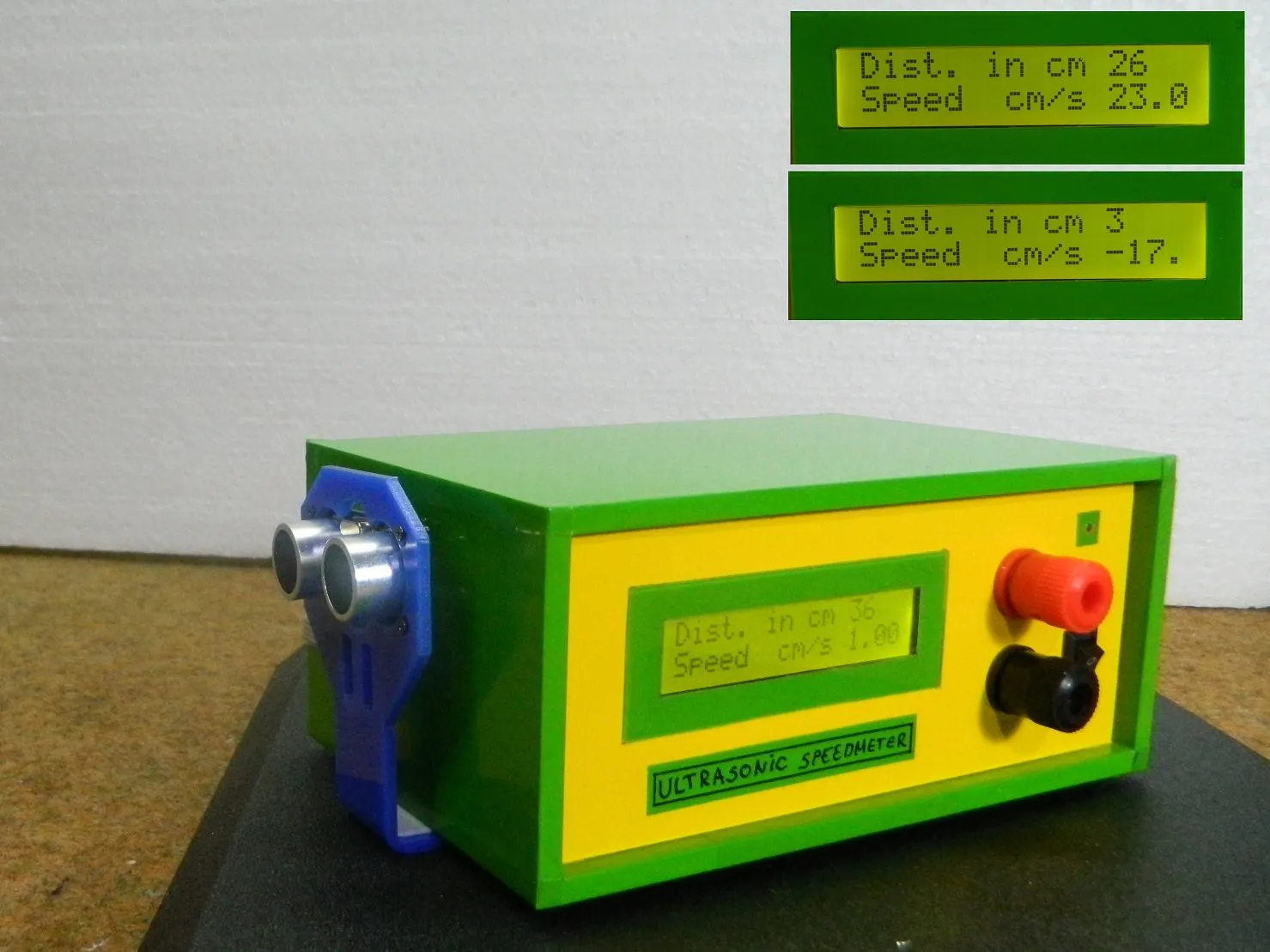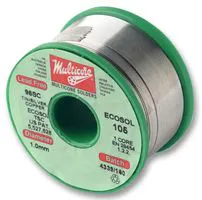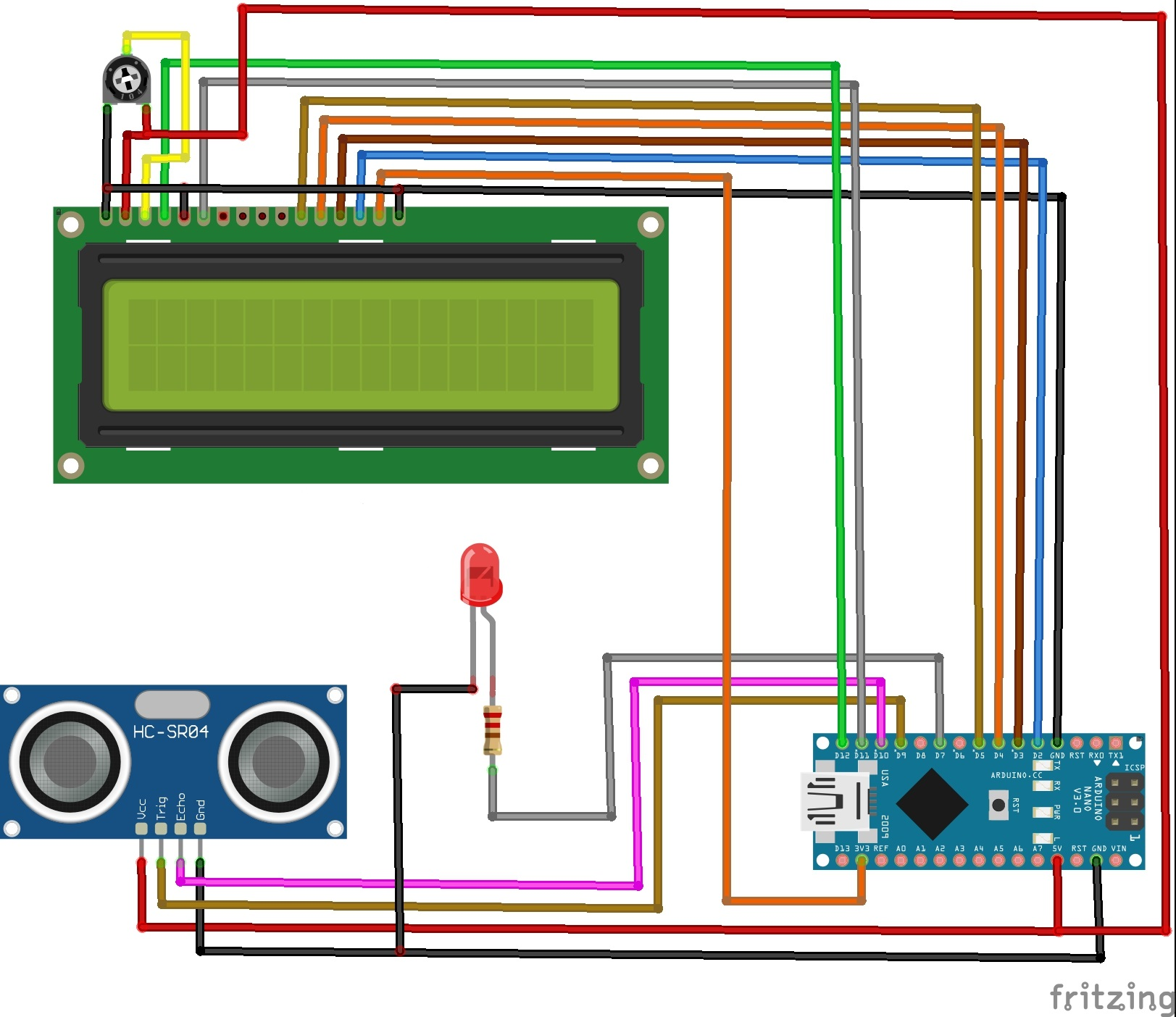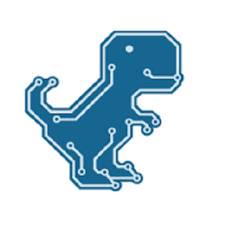Project tutorial# Speed Measurement Using HC-SR04 Ultrasonic Sensor © GPL3+

On this project I will show you how to measure the speed of movement of an object using HC-SR04 ultrasonic sensor.

• 2,151 views
• 0 comments
• 11 respects

## Necessary tools and machinesSoldering iron (generic)Solder Wire, Lead Free

## About this project

HC-SR 04 Ultrasonic sensor is most commonly used to measure distance, but this time I will show you how to measure the speed of movement of an object using this sensor.

Device is very simple and consist only a few components:

- Arduino Nano microcontroller

- LCD display

- Ultrasonic sensor

- and LED diode

For that purpose we need to take two distance measurements in a short time apart and we have:

distance2 - distance1 = distance speed at a given time

If we make the measurements in a time period of 1 second, then we get the speed of movement of the object in cm/s. The basic code is taken from the arduino cc forum and I just added an LCD display for a visual representation of the results.

The first row shows the distance, and the second row shows the speed if the object is moving.

There is also an LED that signals the distance at which the object is placed. If the distance is less than 5 cm, the LED lights up continuously. As the distance increases the LED starts flashing at a speed that depends on the distance of the object. If the object moves away, the blinking is slower, and vice versa.

When the object is moving in the opposite direction, the speed represented on the display has a negative sign.

## Code

##### Arduino codeC/C++
```#include <LiquidCrystal.h>
LiquidCrystal lcd(12, 11, 5, 4, 3, 2);// RS,E,D4,D5,D6,D7
// defines pins numbers
const int trigPin = 9;
const int echoPin = 10;

// defines variables
long duration;
int distance1=0;
int distance2=0;
double Speed=0;
int distance=0;

void setup()
{
lcd.begin(16, 2);// LCD 16X2
pinMode(trigPin, OUTPUT); // Sets the trigPin as an Output
pinMode(echoPin, INPUT); // Sets the echoPin as an Input
pinMode( 7 , OUTPUT);
Serial.begin(9600); // Starts the serial communication
}

void loop()
{

//calculating Speed
distance1 = ultrasonicRead(); //calls ultrasoninicRead() function below

delay(1000);//giving a time gap of 1 sec

distance2 = ultrasonicRead(); //calls ultrasoninicRead() function below

//formula change in distance divided by change in time
Speed = (distance2 - distance1)/1.0; //as the time gap is 1 sec we divide it by 1.

//Displaying Speed
Serial.print("Speed in cm/s  :");
Serial.println(Speed);
lcd.setCursor(0,1);
lcd.print("Speed  cm/s ");
lcd.print(Speed);

// LED indicator
if (distance >0 && distance <5)
{
digitalWrite( 7 , HIGH);
delay(50);                  // waits for a second
}

if (distance > 5 && distance <10 )
{
digitalWrite( 7 , HIGH);
delay(50);                  // waits for a second
digitalWrite( 7 , LOW);    // sets the LED off
delay(50);                  // waits for a second
}

if (distance >10  && distance < 20)
{
digitalWrite( 7 , HIGH);
delay(210);                  // waits for a second
digitalWrite( 7 , LOW);    // sets the LED off
delay(210);                  // waits for a second
}

if (distance >20  && distance < 35)
{
digitalWrite( 7 , HIGH);
delay(610);                  // waits for a second
digitalWrite( 7 , LOW);    // sets the LED off
delay(610);                  // waits for a second
}

}

float ultrasonicRead ()
{
// Clears the trigPin
digitalWrite(trigPin, LOW);
delayMicroseconds(2);

// Sets the trigPin on HIGH state for 10 micro seconds
digitalWrite(trigPin, HIGH);
delayMicroseconds(10);
digitalWrite(trigPin, LOW);

// Reads the echoPin, returns the sound wave travel time in microseconds
duration = pulseIn(echoPin, HIGH);

//calculating distance
distance= duration*0.034/2;

// Prints the distance on the Serial Monitor
Serial.print("Distance in cm : ");
Serial.println(distance);
lcd.setCursor(0,0);
lcd.print("Dist. in cm ");
lcd.print(distance);
lcd.print("   ");
return distance;

}
```

## Schematics## Comments

#### Author##### Mirko Pavleski
• 86 projects
• 474 followers

May 8, 2021

#### Members who respect this project

See similar projects
you might like

#### Ultrasonic Sensor with Alarm, LCD and Temperature

Project tutorial by MichDragstar

• 26,521 views
• 10 comments
• 36 respects

#### Ultrasonic Sensor with LCD Display Using 433MHz

Project tutorial by mcharrison500

• 24,409 views
• 1 comment
• 32 respects

• 26,229 views
• 8 comments
• 41 respects

#### Getting Started with the HC-SR04 Ultrasonic sensor

by Isaac100

• 135,800 views
• 34 comments
• 88 respects

#### Ground Proximity Sensing with Ultrasonic Sensor

Project tutorial by AeroGeeks

• 12,231 views
• 1 comment
• 20 respects

#### Arduino Air Quality Monitor with DSM501A Sensor

Project tutorial by Mirko Pavleski

• 33,241 views
• 3 comments
• 39 respects